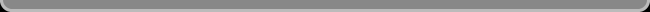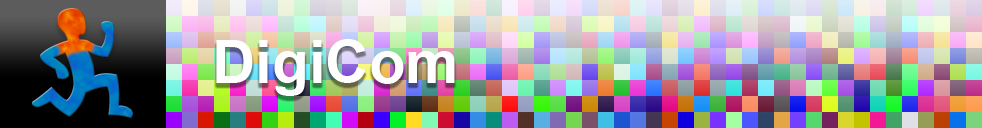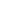Navigation: LaesieWorksImage Compression HomepageThis page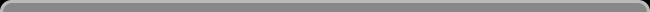OPTICAL CD CODE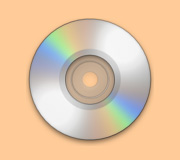This page is about how a common optical medium: the CD, is read (the 120 mm Compact Disc - Digital Audio, Red Book CD from 1982, by Philips & Sony). This page doesn't show you all there is to say about the CD, but it should make clear how to read the code on it.

 Land and Pits Inside a CD is a flat light reflecting layer called "land". On the land are many "pits" written in a spiral-shaped track. From the read lasers point of view, the track spiral goes outwards when the disk rotates counter-clockwise. The spiral has a pitch of 1600 nm Pits are "bumps" really, from the read lasers point of view, because the master disc with pits is duplicated negatively, turning the pits into bumps. Pits have the same light reflecting surface as the land, but pits reflect the read-laser's light in a diffuse and interferening way and thus look relatively dark compaired to the land areas.Data bits and Channel bits It is not simply so that a land is a "1" data bit, and a pit is a "0" data bit. A data bit is a "1" or "0" from the original data, but on a CD there are no data-bits but channel-bits. A channel bit is the smallest time unit used on a CD: 1/4,321,800 sec. a "1" channel bit = a time with change from land to pit, or from pit to land. a "0" channel bit = a time when there is no change. Also; data bytes (8 data bit) are converted into 14 channel bit code with EFM (Eight-to-Fourteen-Modulation), and "glued" with 3 Merging Channel bits before written on the disk. Channel bit length is computed just by dividing the speed by the bit rate. For example: 1.2 m/sec / 4321800 channel bit/sec = 277.662 nm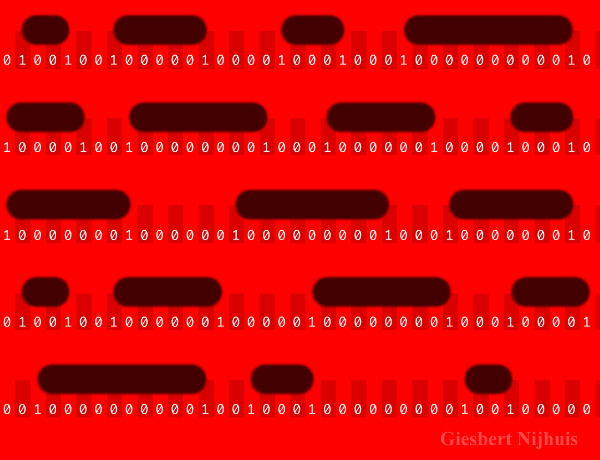Pit & Land Lenght Pit & Land Lenght varies a little depending on how fast the disk turns while recording. ECMA-130 says, "The scanning velocity during recording shall be between 1.20 m/s and 1.40 m/s with a Channel bit rate of 4321800 channel bit/sec. The velocity variation for a disk when recorded shall be within 0.01 m/s" In other words * Discs are recorded at a constant velocity within 0.01/1.3 = 0.8% tolerance. * The actual recording velocity can be between 1.2 and 1.4 m/s, so long as it's constant. * Since the channel bit rate is held constant (4321800 channel bit/sec = 75 blocks/sec * 98 frames/block * 588 channel bits/frame.), then the density of the bits must vary with recording velocity. In other words, those 4321800 channel bits that encode 1 second of audio could be stored in as little as 1.2 linear meters or as much as 1.4 linear meters. The table below shows the size of a channel bit at three different recording velocities, along with the corrsponding sizes of a 3T and 11T pit or land.

 Velocity Channel bit size 3T size 11T size 1.2 m/sec 278 nm 833 nm 3054 nm 1.3 m/sec 301 nm 902 nm 3309 nm 1.4 m/sec 324 nm 972 nm 3563 nmPits and Lands come in nine different lengths, from T3 to T11: T3 = 10001 T4 = 100001 T5 = 1000001 T6 = 10000001 T7 = 100000001 T8 = 1000000001 T9 = 10000000001 T10 = 100000000001 T11 = 1000000000001

 More Pit info Pit width = 500 nm - 600 nm Pit track space = 1600 nm Pit depth = 110 nm - 150 nm. The typically used laser for CD players is an AlGaAs laser diode with a wavelength in air of 780 nm (± 10 nm). The wavelength inside the polycarbonate is a factor of n=1.55 smaller (about 500 nm). The pit is fabricated so that it is a quarter of a wavelength high. The idea here is that light striking the land travels 1/4 + 1/4 = 1/2 of a wavelength further than light striking the top of the pit. The light reflected from the land is then delayed by 1/2 a wavelength, and so is exactly out of phase with the light reflected from the pit. These two waves will interfere destructively, so effectively no light is reflected.

 How the smallest pit is read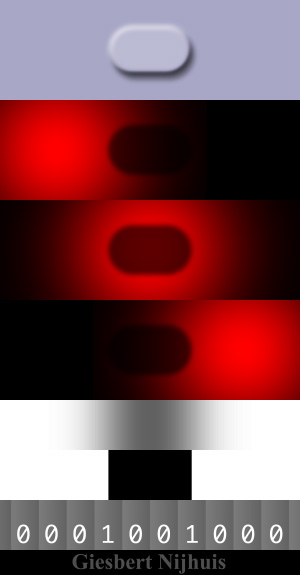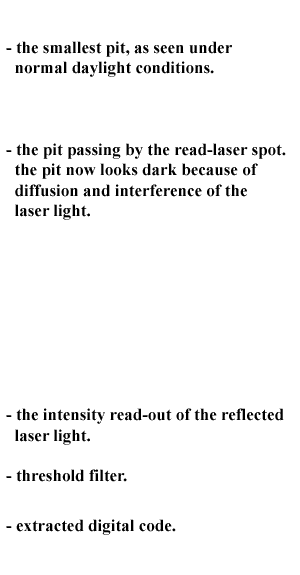EFM (Eight-to-Fourteen-Modulation) EFM is constructed so that each pit/land change is separated by a minimum of two 0's and a maximum of ten 0's. This provides an adequate modulation of signal intensity (sufficient density of pit/land edges encountered) so that the laser tracking mechanism works properly. 8 bit codes are converted in to 14 bit codes: 00000000 becomes 01001000100000 00000001 becomes 10000100000000 click here for all 256 conversions Merging Channel bits Merging Channel bits are three Channel bits, inserted between the bytes of 14 Channel bits as well as between the Sync Header and the adjacent bytes of 14 Channel bits. There are 4 possible merging bit combinations: 000, 001, 010, and 100. The Merging bits shall be chosen such that: 1) the number of ZEROs between consecutive ONEs is everywhere between 2 and 10, 2) the pattern 100000000001000000000010 occurs only at the position for the Sync Header, 3) power spectrum of the HF signal below 20 kHz must be equal to, or below, the spectrum of the minimum system described below. Each group of 14 Channel bits is preceded by three Merging bits to satisfy the requirement of at least two and at most ten ZEROs between two ONEs, also between consecutive groups. Whilst two Merging bits would suffice for this purpose, the third bit is added to be able to minimize the digital sum value (DSV). The DSV at a given position is the sum of the value of the Channel bits from the start of the disk up to the specified position. The DSV must be as close to zero as possible to allow reliable radial tracking and reliable detection of the crossings of HF signals with the decision level. How to write "Nerd" on a CD ASCII character - Decimal code - Binary code - EMF code N = 078 = 01001110 = 00010001000100 e = 101 = 01100101 = 00000000100010 r = 114 = 01110010 = 10010010000010 d = 100 = 01100100 = 01000100100010 EMF code + Merge channel bits: (14 channel bits per byte + 3 Merge channel bits per EMF code) 001 00010001000100 001 00000000100010 000 10010010000010 000 01000100100010 001

 Giesbert Nijhuis